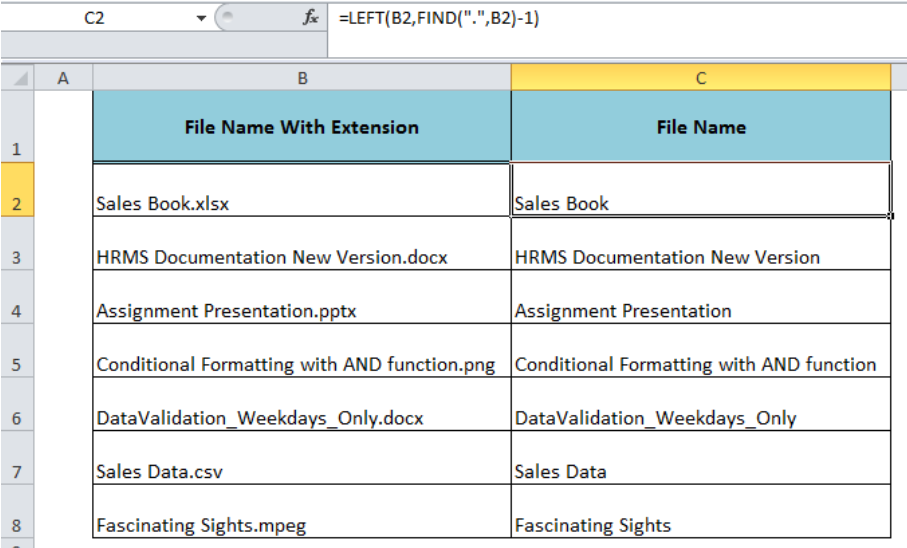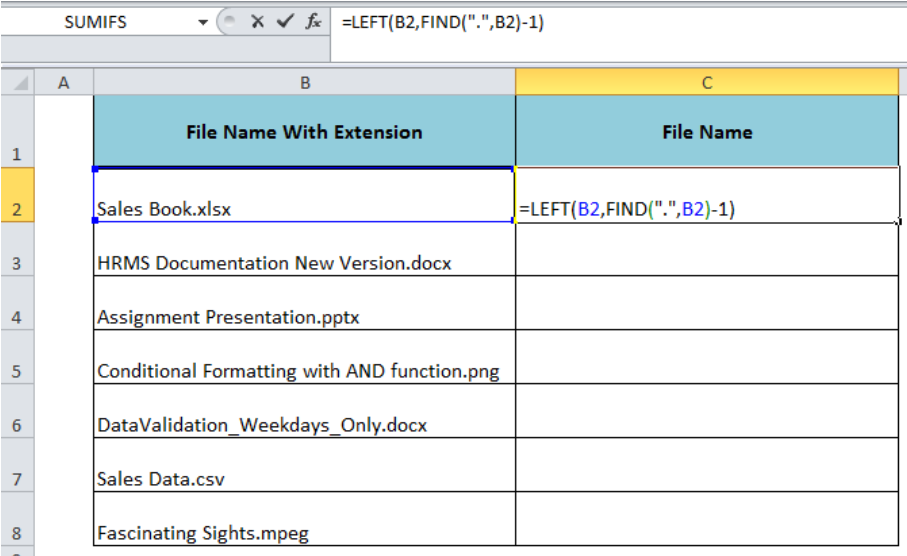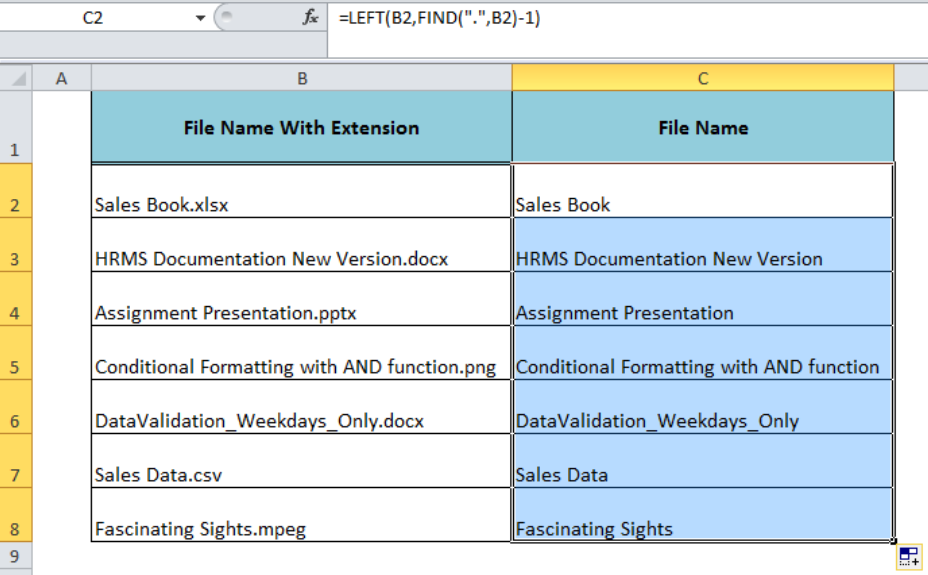Get instant live expert help with Excel or Google Sheets“My Excelchat expert helped me in less than 20 minutes, saving me what would have been 5 hours of work!”

#### Post your problem and you’ll get Expert help in seconds.

Your message must be at least 40 characters
Our professional Expert are available now. Your privacy is guaranteed.

# How to Remove the Extension From Filename in Excel

When we save a filename in an operating system like Microsoft Windows it carries the extension to identify the type of file. The file extension is a string of characters at the end of a filename, usually three or four characters long, that comes after a full stop, like “.doc”, “.xlsx” etc. Using the formula, we can remove the extension from filename in Excel to return the filename only.Figure 1. Removing the Extension From Filename

## Formula Syntax

The syntax for the formula is;

`=LEFT(filename,FIND(".",filename)-1)`

Where,

• Filename – It is a cell reference or text string entered directly as an argument in the formula.
• The LEFT function returns the specified number of characters from the start of the text string.
• The FIND function returns the starting position of a character or one text string within another text string. It is used to determine the number of characters to extract.
• 1 is subtracted from the position of the first match of “.” returned by the FIND function to get the exact number of characters by excluding the “.” from the count.

Note: This formula syntax finds the first match of “.”, therefore it will work perfectly where there are not more than one “.” in the filename.Figure 2. The Syntax For The Formula

## Example

We have a list of filenames that have different file extensions attached with them in column B like “.xlsx”, “.docx”, “.png” etc. and we want to remove the extension from filename returning the filename only in column C. We will use the following formula in cell C2 and drag it down to other cells in column C, such as;

`=LEFT(B2,FIND(".",B2)-1)`Figure 3. The Final Preview of the Formula Result

## Instant Connection to an Expert through our Excelchat Service:

Most of the time, the problem you will need to solve will be more complex than a simple application of a formula or function. If you want to save hours of research and frustration, try our live Excelchat service! Our Excel Experts are available 24/7 to answer any Excel question you may have. We guarantee a connection within 30 seconds and a customized solution within 20 minutes.

### Did this post not answer your question? Get a solution from connecting with the expert.Another blog reader asked this question today on Excelchat:
Solution examplesI need to create a formula that extracts the prefix from column A
Solved by I. Q. in 42 minsi need a formula to combine column y with the number mentioned in column w counting in from 1 to 0 and posting the total count next to number 1 in column v.
Solved by E. J. in 60 minsI would like to insert a formula to add something (Maybe a 'Y') if a cell contains 14 days or less. Is this possible?
Solved by F. H. in 6 minsWhat is the formula for splitting up one column text into 2 columns?
Solved by B. H. in 20 minsI have text and number in multiple cells (Ex: Cell 1: 4S, Cell 2: 3.5A, Cell 3: 8O) and want to sum the number values only (Ex: 4 + 3.5 + 8). How can I do this?
Solved by O. A. in 60 mins## Subscribe to Excelchat.coAnother blog reader asked this question today on Excelchat: